Question

Thermodynamics

1. The volume flow rate is conserved in an incompressible steady flow.

2. In a transient flow process, the rate of change of mass with respect to time is zero.

3. During a steady flow process, the change of energy with respect to time is zero.

4. The temperature of air decreases as it is compressed by an adiabatic compressor.

5. The kinetic energy of a fluid decreases as it passes through an adiabatic diffuser.

In a steady flow device, the mass flow rate of the fluid remains constant.

7. The volume flow rate of air in an adiabatic steady flow compressor remains constant.

The temperature of the fluid remains constant in an adiabatic steady flow process.

There are three mechanisms of energy transfer in a control volume.

10. Air enters a nozzle at a velocity of 30 m/s and leaves at 60 m/s. The change in specific kinetic energy of air in this process is___kJ/kg.

11. Steam enters an adiabatic turbine at 1000 psia and 900°F and leaves as saturated vapor at 5 psia, at a rateof 12.5 Ibm/s. Determine the power output of the turbine, in Btu/s.

2. Argon gas enters an adiabatic turbine steadily at 1600 kPa and 470 °C with a velocity of 55 m/s and leaves at 150 kPa with a velocity of 150 m/s. The inlet area of the turbine is 60 cm?. If the power output of the turbine is 190 kW, determine the exit temperature of the argon.

3. A rigid, insulated tank that is initially evacuated is connected through a valve to a supply line that carries steam at 1.2 MPa and 400°C .Now the valve is opened and the steam is allowed to enter the tank until the pressure reaches 1.2 MPa, at which point the valve is closed. Determine the final temperature of the steam in the tank.

4. Air is compressed steadily in a compressor from 100 kPa and 400 K to 1 MPa and 500 K. The compressor is not well insulated, and a heat loss of 40 kJ/kg occurs during the process. Assuming the changes in kinetic and potential energies are negligible, determine the specific work input to the compressor, in kJ/kg.

5. A hot water stream at 80°C enters a mixing chamber with a mass flow rate of 0.5 kg/s where it is mixed with a stream of cold water at 30°C. If it is desired that the mixture leaves the chamber at 45°C,determine the mass flow rate of the cold-water stream. Assume all the streams are at a pressure of 250kPa.Verified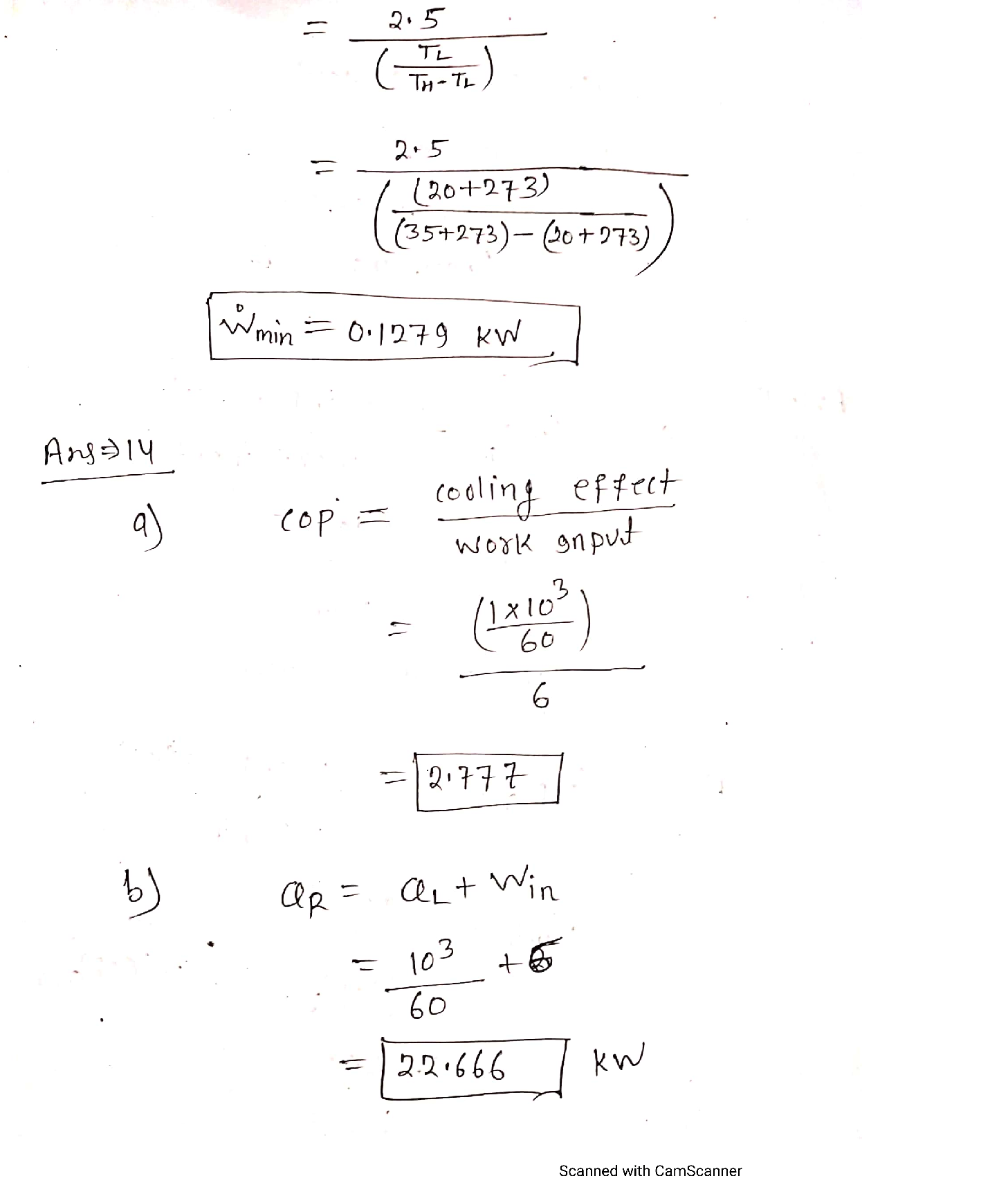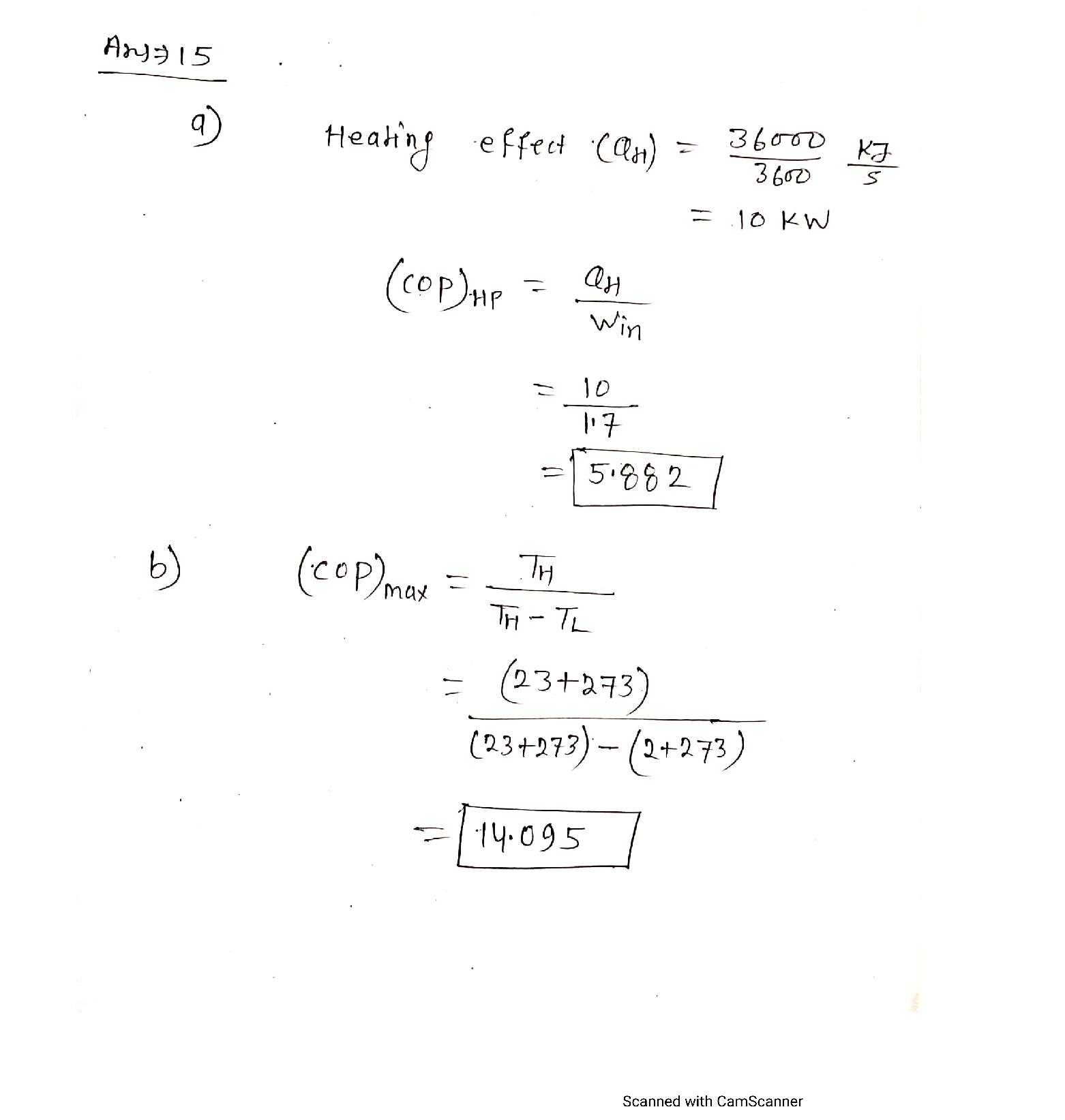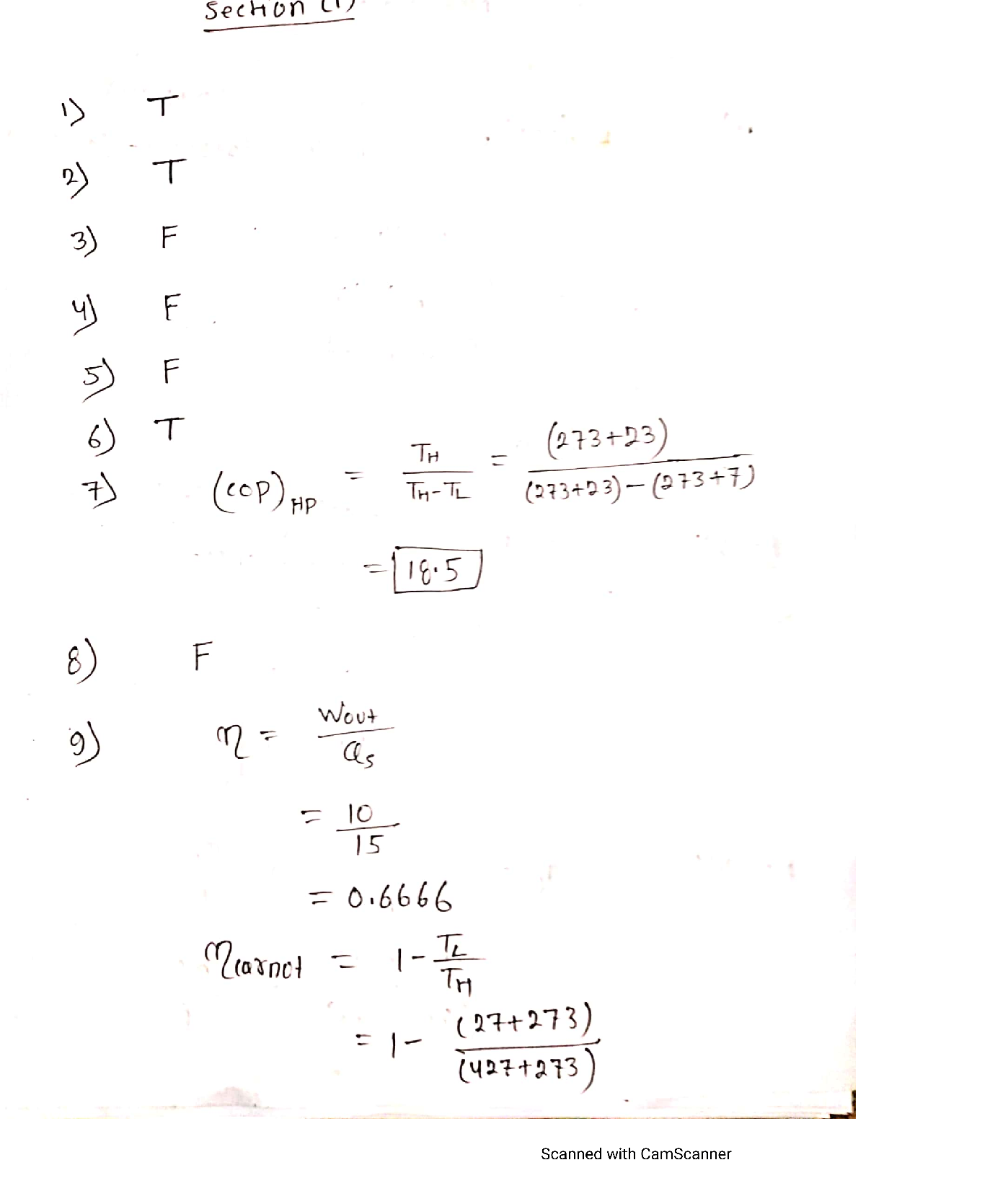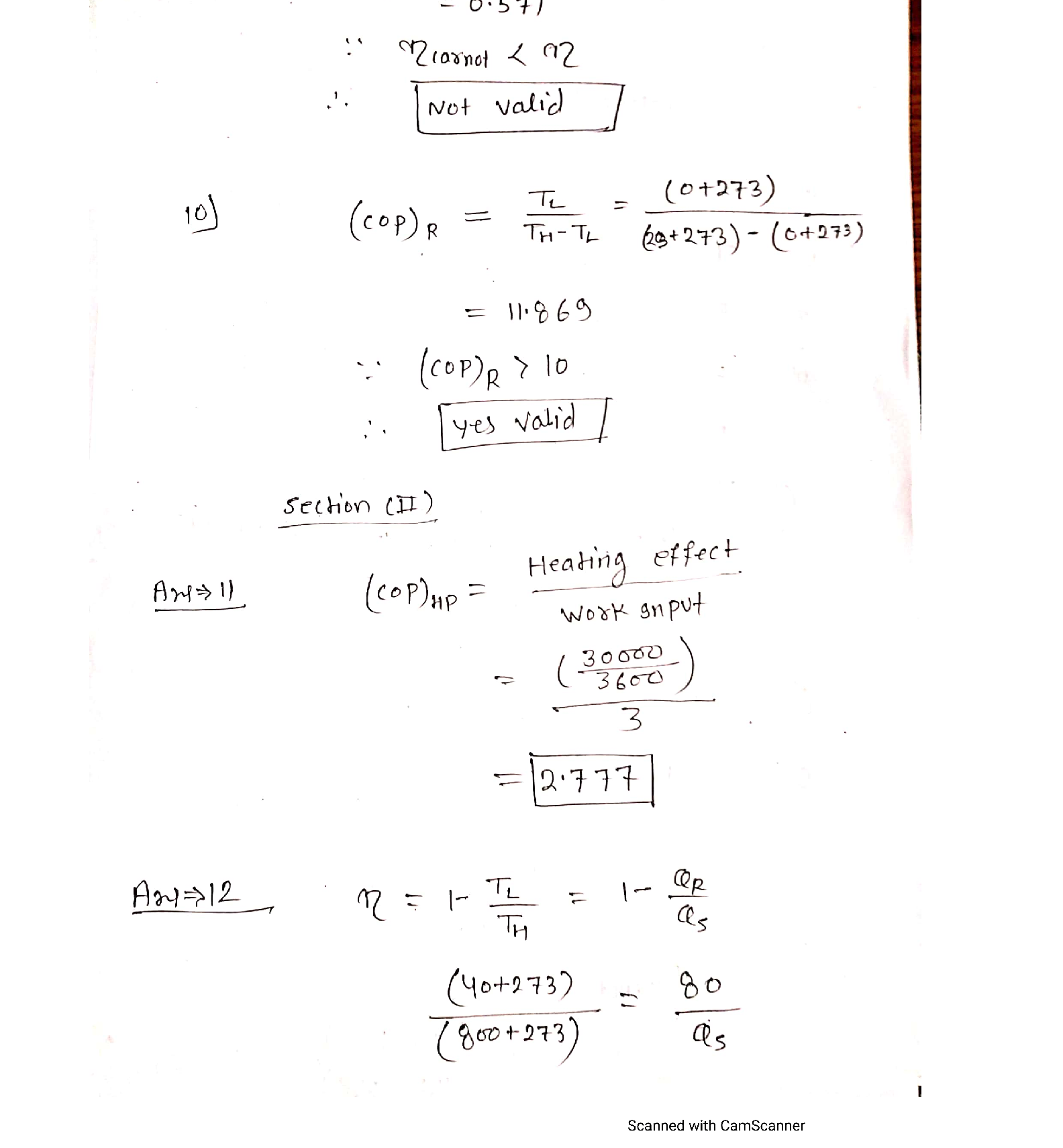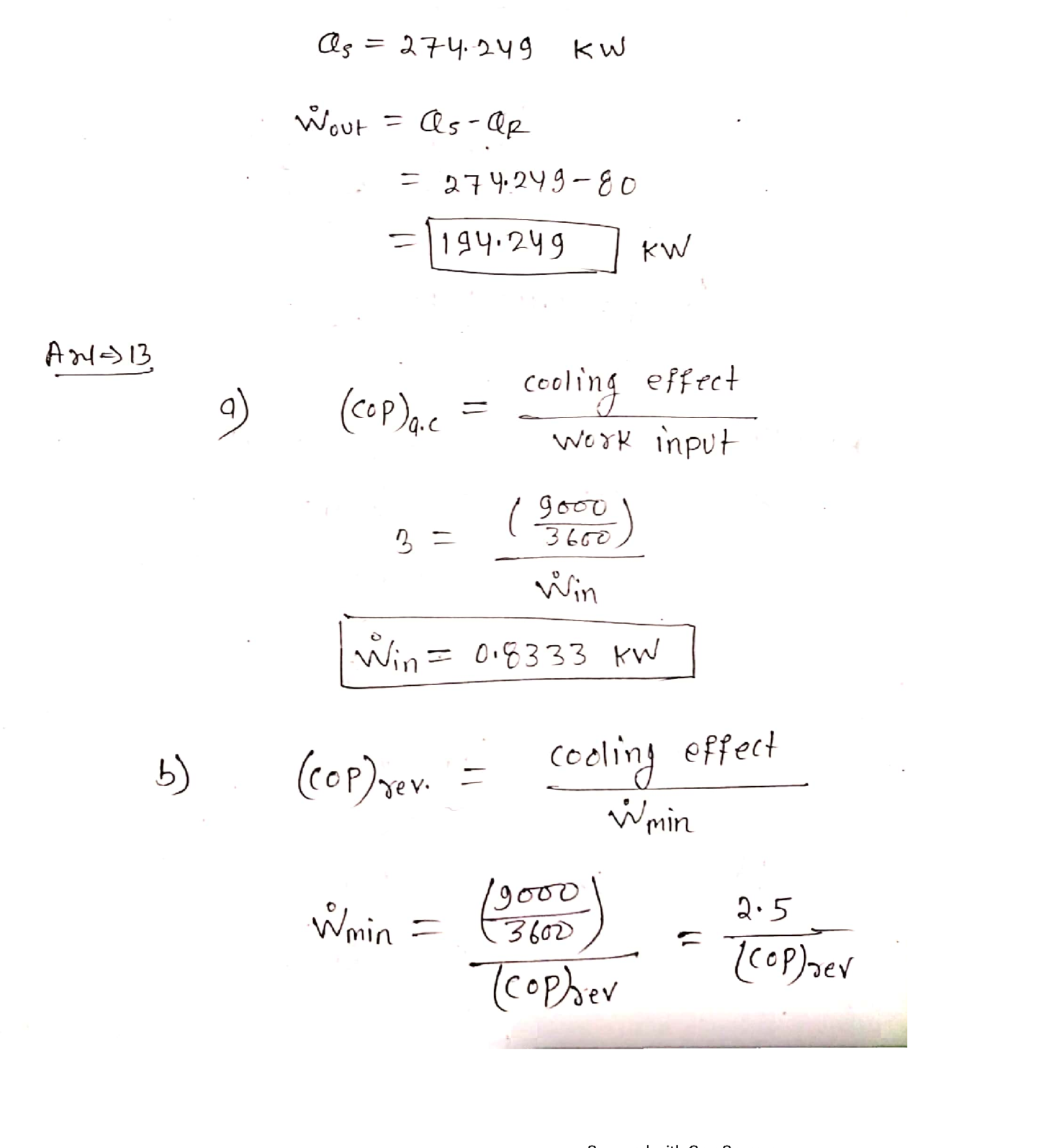### Question 45729Thermodynamics

1. The 2nd law of thermodynamics refers to the quality of energy.
2. Work can convert to heat completely, but heat cannot convert to work completely.
3. The efficiency of a heat engine can be greater than 1.
4. According to Clausius, heat can transfer naturally from cold bodies to hot bodies.
5. The second-law efficiency of a heat engine is always greater than its thermal efficiency.
6. Both the first and second laws of thermodynamics must be satisfied in any real system.
7. The Carnot COP of a heat pump running between 7°C and 23°C is
8. Heat engines with the same 1s* law efficiency will always have the same 2nd law efficiency.
9. An inventor claims to have created a heat engine that produces 10 kW of power for a 15 kW power input, while operating between temperature reservoirs at 27°C and 427°C. Is this claim valid?
10. A manufacturer claims that their refrigerator has a COP of 10 while running between a conditionedspace at 0°C and ambient air at 23°C. Is this claim valid?
11. A heat pump supplies heat to a house at a rate of 30,000 kJ/hr. If the power consumed by the heat pump is 3 kW, determine the coefficient of performance of the heat pump.
12. A Carnot heat engine receives heat from a source at 800°C and rejects heat to a sink at 40°C. The heat is rejected from this engine at a rate of 80 kJ/s. Determine the power output, in kW.
13. An air-conditioner removes heat from a room at 20°C at a rate of 9000 kJ/hr and rejects the heat to the outdoor air at 35°C. If the coefficient of performance of the air-conditioner is 3, determine:
a. The power consumed by the air-conditioner.
b. The minimum power required if the system is reversible.
14. An A/C unit removes heat from the house at a rate of 1 MJ/min. If the required power input to thisA/C unit is 6 kW, determine:
COP of the air-conditioning unit.
b. The rate of heat rejection to the outside air, in kW.
15. A heat pump absorbs heat from the cold outdoors at 2° C and supplies heat to a house at 23° C at a rate of 36,000 kJ/hr.
a. What is the COP of the heat pump if the power consumed is 1.7 kW?
b. What is the maximum COP of the heat pump?

### Question 45728Thermodynamics

1. The volume flow rate is conserved in an incompressible steady flow.
2. In a transient flow process, the rate of change of mass with respect to time is zero.
3. During a steady flow process, the change of energy with respect to time is zero.
4. The temperature of air decreases as it is compressed by an adiabatic compressor.
5. The kinetic energy of a fluid decreases as it passes through an adiabatic diffuser.
In a steady flow device, the mass flow rate of the fluid remains constant.
7. The volume flow rate of air in an adiabatic steady flow compressor remains constant.
The temperature of the fluid remains constant in an adiabatic steady flow process.
There are three mechanisms of energy transfer in a control volume.
10. Air enters a nozzle at a velocity of 30 m/s and leaves at 60 m/s. The change in specific kinetic energy of air in this process is___kJ/kg.
11. Steam enters an adiabatic turbine at 1000 psia and 900°F and leaves as saturated vapor at 5 psia, at a rateof 12.5 Ibm/s. Determine the power output of the turbine, in Btu/s.
2. Argon gas enters an adiabatic turbine steadily at 1600 kPa and 470 °C with a velocity of 55 m/s and leaves at 150 kPa with a velocity of 150 m/s. The inlet area of the turbine is 60 cm?. If the power output of the turbine is 190 kW, determine the exit temperature of the argon.
3. A rigid, insulated tank that is initially evacuated is connected through a valve to a supply line that carries steam at 1.2 MPa and 400°C .Now the valve is opened and the steam is allowed to enter the tank until the pressure reaches 1.2 MPa, at which point the valve is closed. Determine the final temperature of the steam in the tank.
4. Air is compressed steadily in a compressor from 100 kPa and 400 K to 1 MPa and 500 K. The compressor is not well insulated, and a heat loss of 40 kJ/kg occurs during the process. Assuming the changes in kinetic and potential energies are negligible, determine the specific work input to the compressor, in kJ/kg.
5. A hot water stream at 80°C enters a mixing chamber with a mass flow rate of 0.5 kg/s where it is mixed with a stream of cold water at 30°C. If it is desired that the mixture leaves the chamber at 45°C,determine the mass flow rate of the cold-water stream. Assume all the streams are at a pressure of 250kPa.

### Question 45713Thermodynamics

A brine pipe is connected to the bottom of a tank containing Mercury, Water, and Air through atube as shown in the figure below.
When a 0.9 kPa air pressure change occurs, while the pressure in the brine pipe remains constant,the liquid interface between brine and mercury in the right tube drops a distance of 5 mm.
\text { a) Provide a relation between air pressure and brine pipe pressure. }
b) Schematize the system showing the initial and final levels of the brine-mercury interface.
c) What is the new relation between air pressure and brine pipe pressure?
d) Determine the ratio of the tube cross-section to the tank cross-section (A2/A1).

### Question 45712Thermodynamics

The figure below represents the T-v diagram of the expansion of an ideal gas in a piston-cylinder system.
Can we use this plot to graphically determine the work done? Explain your answer providing all necessary details.

### Question 45711Thermodynamics

Kinetic energy and flow energy are two types of energy that a system can possess that are both related to fluid motion. Define each of them and concisely explain the difference between the two.

### Question 45673Thermodynamics

A siphoning process is used to empty the water out of a fish tank in order to clean it. Figure C.2 shows a diagram of the setup. The hose outlet (point 2) is located 1.10 m below the water level in the tank (point 1) and highest position of the hose (point 3) is located at 0.25 m above this point. The siphon hose used has an internal diameter of 6 mm.
(i)Velocity of the water at the hose outlet using Bernoulli's equation.
(ii)Mass flow rate of the siphon water.
(iii)Time needed to siphon 80 litres of water.
(iv)Pressure at the highest position of the siphon hose (point 3).

### Question 45672Thermodynamics

A horizontal jet of water strikes a curved vane as shown in Figure C.1. The external angle of the curved vane is 158°.The mean velocity and volumetric flow rate of the water jet at position 1 are 12 m/s and 55 m³/h respectively. Dueto friction, the water jet leaves the vane at position 2 with 92 % its original velocity.
(i)Direct force exerted by the water jet on the vane in the x - direction.
(ii)Direct force exerted by the water jet on the vane in the y - direction.
(ii)Net resultant direct force and angle on the vane.

### Question 45671Thermodynamics

A dam has a cross sectional profile with its water face made up of two inclined slopes as shown in Figure A.2. Thedepth of the water is 11 m, and the span of the dam is 100 m.
(i)Draw a fully annotated schematic of the front and side views of the dam showing all the relevant depth sand hydrostatic forces.
(ii)Horizontal force component acting on the dam.
(iii)Depth from water free surface where the horizontal force component acts on the dam.
(iv)Vertical force component acting on the dam.
(v)Resultant force acting on the dam and angle from the horizon at which the resultant force acts.

### Question 45670Thermodynamics

A mixture of gases has a temperature and pressure of 300 K and 470 kPa respectively. A gravimetric analysis performed on the mixture gave the proportions as shown below in Table B.2-1.
(i)Mass fractions and molar masses for each of the mixture's constituent gases.
(ii)Volume fractions of the constituent gases in the mixture.
(ii)Partial pressures of the constituent gases in the mixture.
(iv)Mean molar mass and specific gas constant for the mixture of gases.
(v)Specific heat capacity at constant pressure and specific heat capacity at constant volume for the mixture of gases.

### Question 45669Thermodynamics

Oxygen in piston and cylinder system is at initial state of 600 kPa and 500 K (state 1). It has a specific gas constant,R, of 259.8 J/(kg K) and specific heat capacity at constant pressure, Cp, of 1031 J/(kg K). The oxygen expands with constant volume ratio, v2/v1, of 2.3. The three different thermodynamic expansion processes (from the same initial state) to be investigated are: (a) Isobaric, (b) Isothermal, and (c) Isentropic.
(i)Calculate the specific volume at the initial state, v,, the specific heat capacity at constant volume, Cy, and the ratio of specific heat capacities, y.
(ii)Determine the pressure, specific volume, and temperature at the final state for the different processes.Show all calculations and summarise the answers in a table like Table B.1-1 below.
Determine the specific heat transfer, specific work transfer, and change in specific internal energy for the different processes. Show all calculations and summarise the answers in a table like Table B.1-2 below.
State all assumptions used in the above analysis.
Sketch a full annotated pressure vs. specific volume diagram for the oxygen at the initial state undergoing the three different expansion processes.

### Submit query